Important Triangle Questions

Chapter 7 Class 9 Triangles
Serial order wise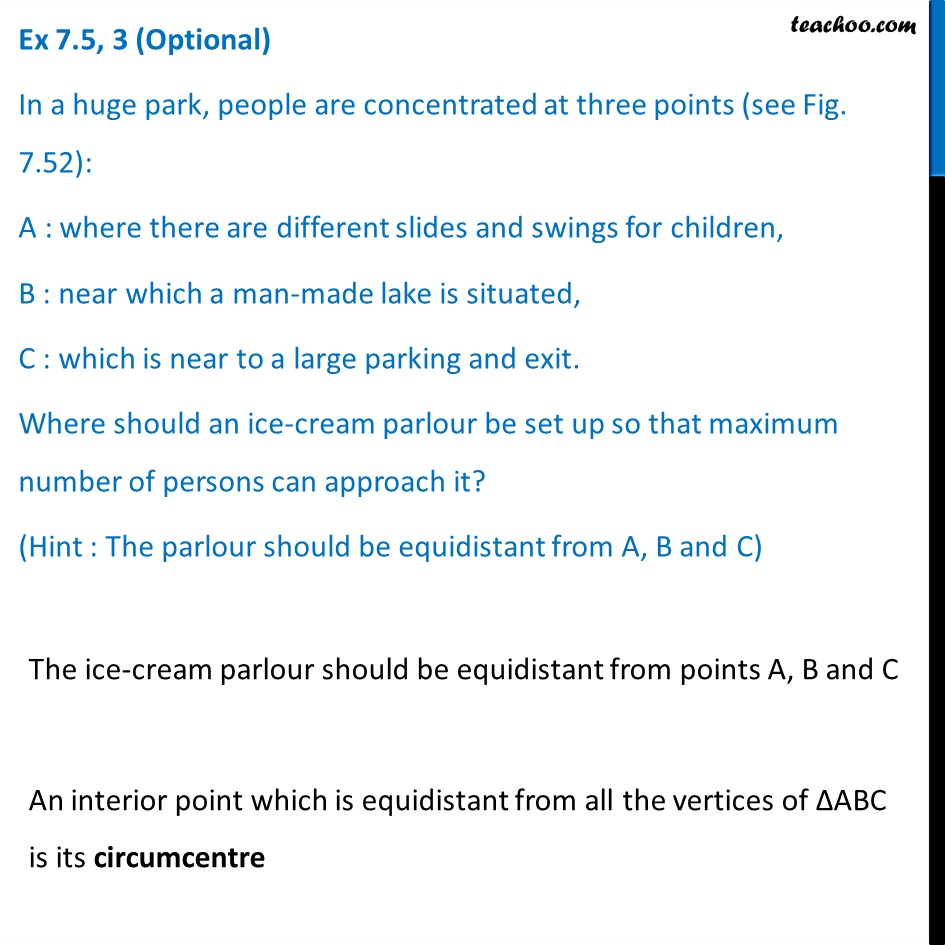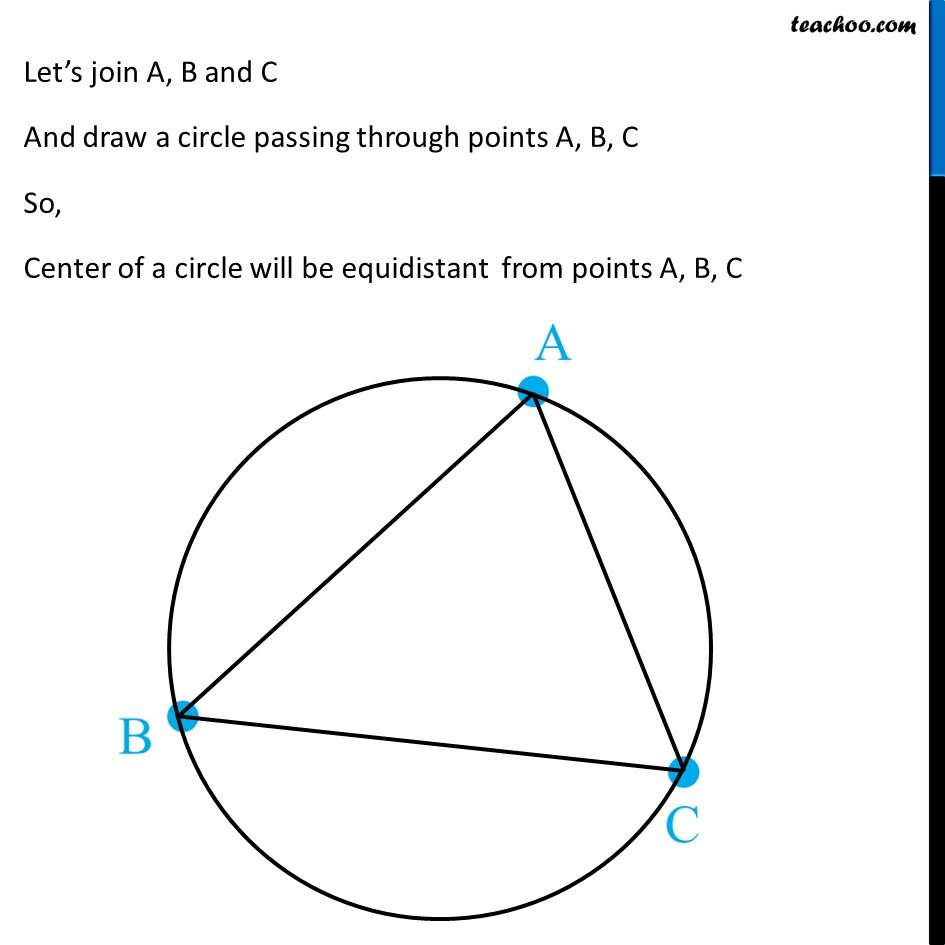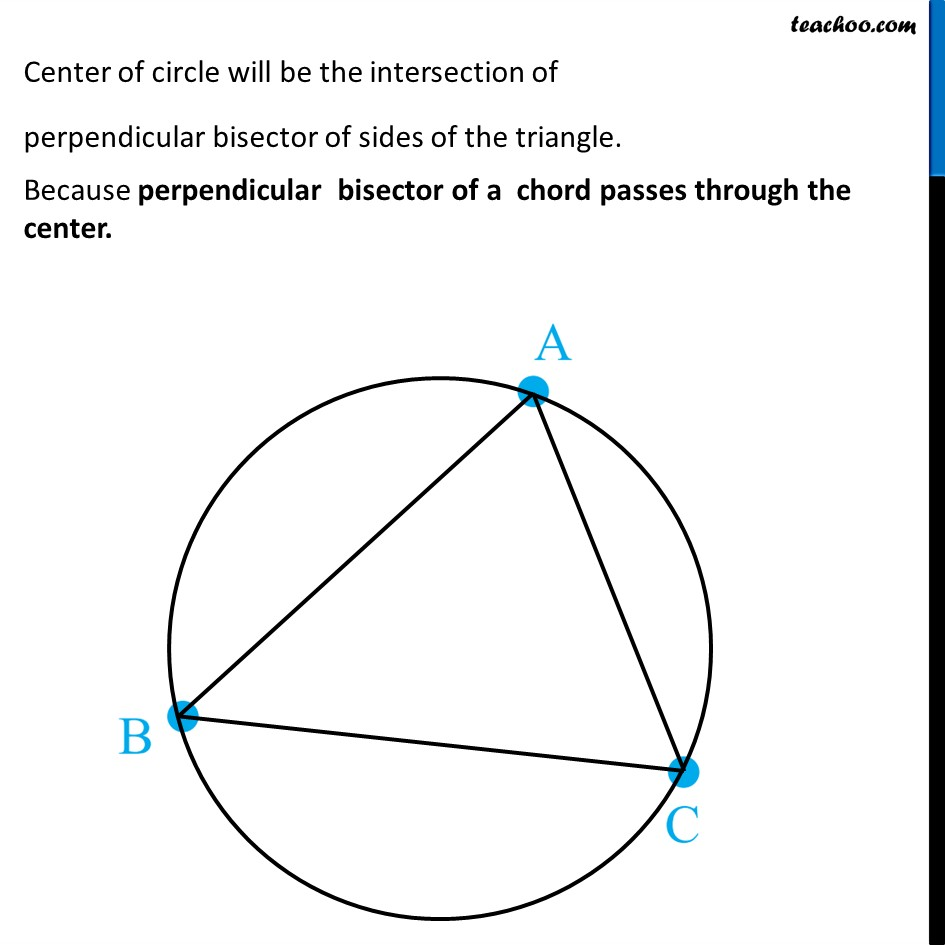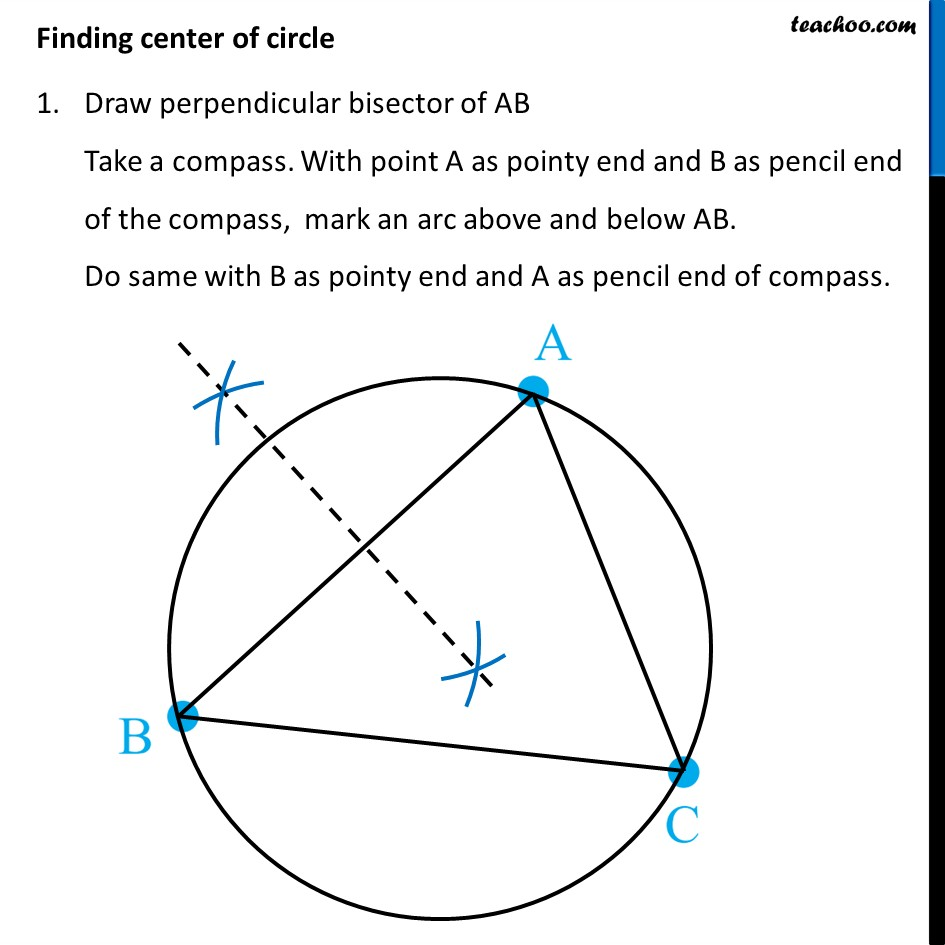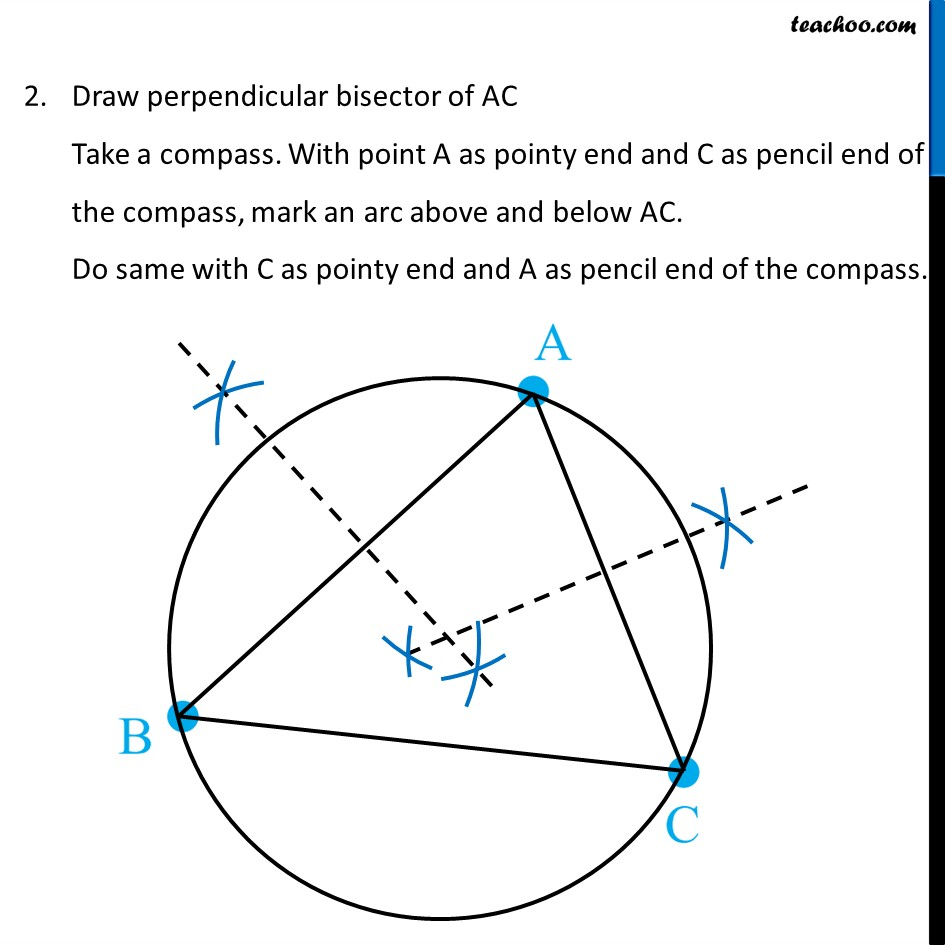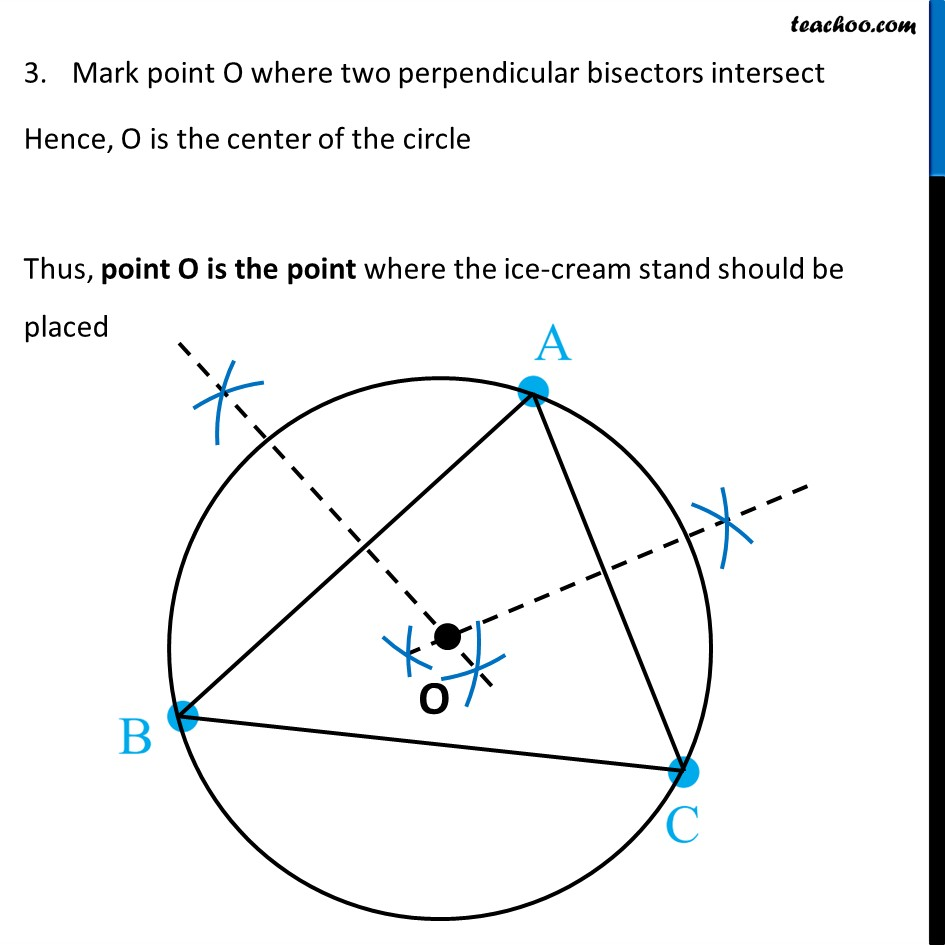Learn in your speed, with individual attention - Teachoo Maths 1-on-1 Class

### Transcript

Question 3 In a huge park, people are concentrated at three points (see Fig. 7.52): A : where there are different slides and swings for children, B : near which a man-made lake is situated, C : which is near to a large parking and exit. Where should an ice-cream parlour be set up so that maximum number of persons can approach it? (Hint : The parlour should be equidistant from A, B and C) The ice-cream parlour should be equidistant from points A, B and C An interior point which is equidistant from all the vertices of ∆ABC is its circumcentre Let’s join A, B and C And draw a circle passing through points A, B, C So, Center of a circle will be equidistant from points A, B, C Center of circle will be the intersection of perpendicular bisector of sides of the triangle. Because perpendicular bisector of a chord passes through the center. Finding center of circle Draw perpendicular bisector of AB Take a compass. With point A as pointy end and B as pencil end of the compass, mark an arc above and below AB. Do same with B as pointy end and A as pencil end of compass. Mark point O where two perpendicular bisectors intersect Hence, O is the center of the circle Thus, point O is the point where the ice-cream stand should be placed